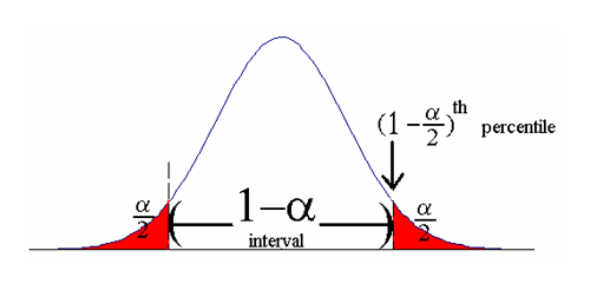# Confidence Intervals Assessment Test

10 Questions | Total Attempts: 296SettingsConfidence intervals are used in statistics as a type of interval estimate that is computed from the observed data. Take this assessment test to evaluate your knowledge of confidence intervals.

• 1.
Which is a type of estimate for population parameter?
• A.

Shore

• B.

Super

• C.

Off estimate

• D.

Point estimate

• 2.
The null value of the confidence interval for the relative risk is...
• A.

0

• B.

1

• C.

2

• D.

15

• 3.
Which is an example of dichotomous variable?
• A.

Estimate

• B.

Portion

• C.

Density

• D.

Population proportion

• 4.
A range of values that is likely to contain the value of an unknown population parameter is...
• A.

Density

• B.

Linear

• C.

Discreet

• D.

Confidence interval

• 5.
Which is an important parameter in calculating confidence interval?
• A.

Mean

• B.

Ratio

• C.

Time

• D.

Level

• 6.
What is the confidence interval for 99?
• A.

1.01

• B.

2.58

• C.

3.89

• D.

0.66

• 7.
What is the z value for 99 confidence interval?
• A.

3.33

• B.

0.06

• C.

2.58

• D.

1.88

• 8.
Wide confidence intervals mean that your sample size was too...
• A.

Huge

• B.

Small

• C.

Big

• D.

Wide

• 9.
What is the critical value for 95 percent confidence interval?
• A.

1

• B.

0

• C.

0.88

• D.

1.96

• 10.
A statistic expressing the amount of random sampling error in a survey's results is...
• A.

Curve

• B.

Line

• C.

Margin of error

• D.

Error of slope

Related Topics